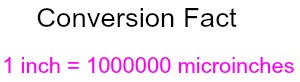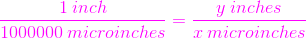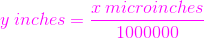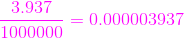# Converting Microinches to Inches

Instructor: Laura Pennington

Laura received her Master's degree in Pure Mathematics from Michigan State University, and her Bachelor's degree in Mathematics from Grand Valley State University. She has 20 years of experience teaching collegiate mathematics at various institutions.

In this lesson, we will learn how to convert microinches to inches in one very easy step. Then we'll look at two different applications of microinches to see how converting to inches can give us a clearer picture.

## Steps to Solve

When it comes to converting microinches to inches, it's really very simple. The whole process of conversion is based on the fact that 1 inch = 1,000,000 microinches.Wow! That's extremely small - way too small to detect with the human eye, but back to this conversion! We can use proportions to illustrate how to convert microinches to inches. Suppose we want to convert x microinches to inches. We don't know how many inches this is, so we'll just say that x microinches is y inches. This tells us that we want to find y.

So we know that y inches = x microinches, and that 1 inch = 1000000 microinches. Therefore, if we divide 1 inch / 1000000 microinches, we get 1, and if we divide y inches by x microinches, we get 1. So 1 inch / 1000000 microinches = y inches / x microinches. This gives us the following proportion:Now recall that we want to solve for y, because we want to convert microinches to inches. If we cross multiply in the above proportion, we get the following:

x = 1000000y

Now, we can easily solve for y in terms of x by dividing both sides by 1000000:

y = x / 1000000

If you're thinking that all of this seems like a lot of work to just convert microinches to inches, you are correct! The fact of the matter is that from what we just saw, we know that:

y inches = x microinches / 1000000

This tells us that to convert x microinches to inches, we simply divide by 1000000. For example, say we wanted to convert 350 microinches to inches. All we have to do is divide 350 by 1000000.

350 / 1000000 = 0.00035

We find that 350 microinches = 0.00035 inches. Easy-peasy, right?

## Solution

To convert from microinches to inches, use the relationship between inches and microinches, that 1 inch = 1000000 microinches.Because of this relationship, we find that converting from microinches to inches is simply a matter of dividing the number of microinches by 1000000.## Applications of Microinches

Now we know how to convert microinches to inches, but with this measurement being so small, you may wonder how there would ever be a use for it. You would be surprised! Microinches can be used in medicine, engineering, entomology, and many other areas.

In medicine, very tiny bacteria, viruses, and other organisms are studied, many of which are too small to detect with the human eye. If we could see viruses and bacteria with our eyes, we would be much less likely to get a cold or the flu, because we would simply wash the virus off of our hands before it had a chance to make contact with a mucus membrane. To put this into perspective, let's consider an example: On average, the flu virus is about 3.937 microinches in diameter. To convert this to inches, we divide by 1000000 to get 3.937 / 1000000 = 0.000003937.This tells us that on average, the flu virus is 0.000003937 inches in diameter. That's so tiny! There's no way we could detect that with our eyes. No wonder it's so important to wash your hands often during cold and flu season!

To unlock this lesson you must be a Study.com Member.

### Register to view this lesson

Are you a student or a teacher?